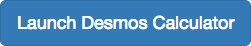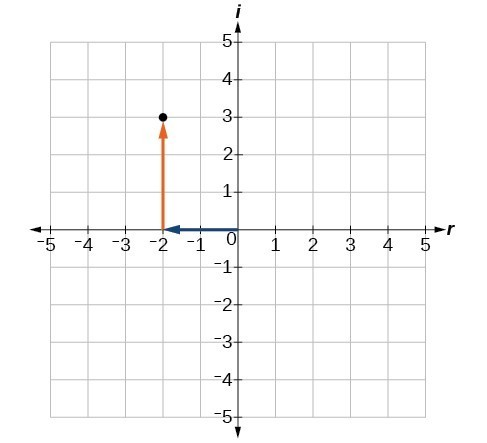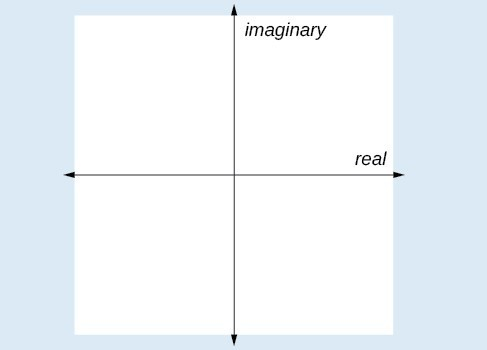## Express and Plot Complex Numbers

### Learning Outcomes

• Express square roots of negative numbers as multiples of $i$.
• Plot complex numbers on the complex plane.We know how to find the square root of any positive real number. In a similar way we can find the square root of a negative number. The difference is that the root is not real. If the value in the radicand is negative, the root is said to be an imaginary number. The imaginary number $i$ is defined as the square root of negative 1.

$\sqrt{-1}=i$

${i}^{2}={\left(\sqrt{-1}\right)}^{2}=-1$

We can write the square root of any negative number as a multiple of $i$. Consider the square root of –25.

\begin{align}\sqrt{-25}&=\sqrt{25\cdot \left(-1\right)}\\&=\sqrt{25}\cdot\sqrt{-1}\\ &=5i\end{align}

We use $5i$ and not $-\text{5}i$ because the principal root of 25 is the positive root.

A complex number is the sum of a real number and an imaginary number. A complex number is expressed in standard form when written $a+bi$ where $a$ is the real part and $bi$ is the imaginary part. For example, $5+2i$ is a complex number. So, too, is $3+4\sqrt{3}i$.Imaginary numbers are distinguished from real numbers because a squared imaginary number produces a negative real number. Recall, when a positive real number is squared, the result is a positive real number and when a negative real number is squared, again, the result is a positive real number. Complex numbers are a combination of real and imaginary numbers.

### A General Note: Imaginary and Complex Numbers

A complex number is a number of the form $a+bi$ where

• $a$ is the real part of the complex number.
• $bi$ is the imaginary part of the complex number.

If $b=0$, then $a+bi$ is a real number. If $a=0$ and $b$ is not equal to 0, the complex number is called an imaginary number. An imaginary number is an even root of a negative number.

### How To: Given an imaginary number, express it in standard form.

1. Write $\sqrt{-a}$ as $\sqrt{a}\cdot\sqrt{-1}$.
2. Express $\sqrt{-1}$ as i.
3. Write $\sqrt{a}\cdot i$ in simplest form.

### Example: Expressing an Imaginary Number in Standard Form

Express $\sqrt{-9}$ in standard form.

### Try It

Express $\sqrt{-24}$ in standard form.

## Plot complex numbers on the complex plane

We cannot plot complex numbers on a number line as we might real numbers. However, we can still represent them graphically. To represent a complex number we need to address the two components of the number. We use the complex plane, which is a coordinate system in which the horizontal axis represents the real component and the vertical axis represents the imaginary component. Complex numbers are the points on the plane, expressed as ordered pairs $(a, b)$, where $a$ represents the coordinate for the horizontal axis and $b$ represents the coordinate for the vertical axis.Let’s consider the number $-2+3i$. The real part of the complex number is $–2$ and the imaginary part is $3i$. We plot the ordered pair $\left(-2,3\right)$ to represent the complex number $-2+3i$.

### A General Note: Complex PlaneIn the complex plane, the horizontal axis is the real axis, and the vertical axis is the imaginary axis.

### How To: Given a complex number, represent its components on the complex plane.

1. Determine the real part and the imaginary part of the complex number.
2. Move along the horizontal axis to show the real part of the number.
3. Move parallel to the vertical axis to show the imaginary part of the number.
4. Plot the point.

### Example: Plotting a Complex Number on the Complex Plane

Plot the complex number $3 - 4i$ on the complex plane.

### Try It

Plot the complex number $-4-i$ on the complex plane.

## Contribute!

Did you have an idea for improving this content? We’d love your input.